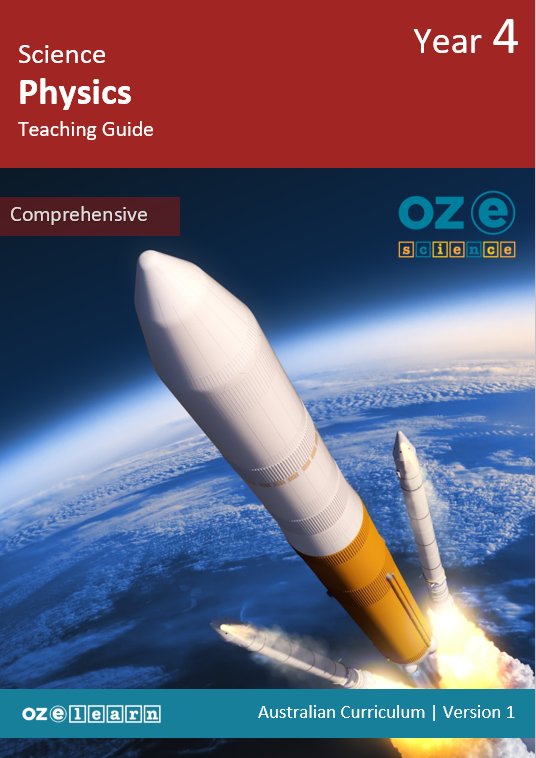# Oz-e-science

Physics Comprehensive - Year 4### Extra resources

#### Overview

Physics Comprehensive Year 4 is a Physical Sciences curriculum program for Year 4 students. It aligns to the Australian Curriculum Science Understanding:
• Forces can be exerted by one object on another through direct contact or from a distance (ACSSU076).

Note: This curriculum program is currently being updated to align with the Australian Curriculum 9.0. Updated versions will be released in early 2024.
Learning Objectives
In Lessons 1 to 15, students learn about:
• what a force is and identify some common examples of forces in our everyday lives
• identifying different types of forces and explain the difference between contact and non-contact forces
• how to describe the ways forces can push and pull objects, causing them to move or stop moving
• how forces can make objects speed up and slow down
• how forces can resist objects when they are moving
• how forces can stretch objects and what happens when that force is released
• how forces can repel two objects away from each other or attract two objects closer to each other
• the force of gravity and how it affects objects of different shapes and sizes
• how size and direction of forces can move and change objects
• Sir Isaac Newton and his First Law of Forces
• Newton’s Second Law of Motion
• Newton’s Third Law of Motion
• using the Scientific Method to investigate Newton’s laws
• using the Scientific Method to evaluate and communicate the results of the experiment.
Success Criteria
• Describe what a force is.
• Identify some common examples of forces.
• Explain what happens to an object when a force acts on it.
• Identify contact and non-contact forces.
• Explain what happens to objects when contact and non-contact forces act on them.
• Identify the forces used in pushing and pulling objects.
• Investigate motion by experimenting with pushing and pulling objects.
• Identify the forces used in speeding up and slowing down objects.
• Explain what acceleration and deceleration are.
• Investigate motion by experimenting with speeding up and slowing down objects.
• Identify the forces that can resist an object’s movement.
• Explain what friction and resistance are.
• Investigate friction by experimenting with objects moving.
• Identify the forces that can stretch objects.
• Explain what being elastic means.
• Investigate stretch by experimenting with applying force to objects.
• Identify the forces that can attract and repel objects.
• Explain what magnetic fields are.
• Investigate attracting and repelling by experimenting with magnets.
• Explain what gravity is.
• Describe how gravity works.
• Investigate gravity by experimenting with objects falling.
• Compare the size and direction of forces.
• Describe what happens to an object experiencing more than one force.
• Investigate forces by creating a marble rollercoaster.
• Explain who Isaac Newton is.
• Describe what Newton’s First Law is.
• Investigate Newton’s First Law by experimenting with moving objects.
• Explain Newton’s Second Law of Motion.
• Describe what mass is.
• Investigate Newton’s Second Law by experimenting with falling objects.
• Explain Newton’s Third Law of Motion.
• Describe what a force pair is.
• Investigate Newton’s Third Law by experimenting with pushing objects.
• Identify the steps in the Scientific Method.
• Experiment with Newtons laws using cars on a ramp.
• Measure the effect that steepness of a ramp has on forces.
• Create a scientific question.
• Create a hypothesis for an experiment.
• Predict the outcome of an experiment.
• Plan an experiment.
• Measure the effect that mass of an object has on forces.
• Evaluate the results of an experiment.
• Explain the findings using Newton’s laws.
• Communicate findings to the class.
Assessment
Progress Tests
Progress tests are conducted after every second lesson, allowing teachers to monitor student understanding of the concepts taught over the past two lessons and to identify where reteaching is needed. The Teaching Guide contains the testing questions, and the Student Workbook has a section where students write their answers and score themselves.

Structured Research Activity
The Structured Research Activity (SRA) for this unit is: Students plan and conduct an experiment to measure the effect that mass of an object has on forces and report findings to the class. The SRA takes place over two lessons so students can apply the Science Understanding and Science Inquiry Skills covered in the unit. Teachers use the Guide to Making Judgements, which is included in the Teaching Guide, to mark the SRA.

End-of-Unit Assessment
The last lesson is the end-of-unit assessment, which has a variety of question formats (e.g. label the diagram, circle the correct answer) to assess student mastery of content from the unit. The end-of-unit assessment is in the Teaching Guide. Teachers copy the assessment and distribute to students at testing time.
Explicit Instruction For Science
Oz-e-science applies a teaching approach and curriculum that uses explicit teaching of content and knowledge of science as well as explicit teaching and practical scientific inquiry using the Scientific Method. Read more about pedagogy here.

## Recommended Units

\Learnworlds\Codeneurons\Pages\ZoneRenderers\CourseCards